# Numerical Investigation of Magneto-Hydrodynamics Mixed Convection in a Square Cavity for Various Shaped Conducting Obstacles Placed at the Center

Numerical Investigation of Magneto-Hydrodynamics Mixed Convection in a Square Cavity for Various Shaped Conducting Obstacles Placed at the Center

Md. Feroz AlamMayur Krishna Bora Bishwajit Sharma Rabindra Nath Barman

Department of Mechanical Engineering, National Institute of Technology Durgapur, India

Corresponding Author Email:
rn.barman@me.nitdgp.ac.in
Page:
550-556
|
DOI:
https://doi.org/10.18280/mmep.060410
11 January 2019
|
Accepted:
11 November 2019
|
Published:
24 December 2019
| Citation

OPEN ACCESS

Abstract:

A numerical study of mixed convection in a square enclosure from a heating element to a cold moving wall is performed under the isothermal heating conditions. The heater is placed at the bottom boundary and the cold upper wall moves horizontally towards right side with constant velocity. The lateral walls are insulated. The study includes the thermal performance of nanofluid for various shaped obstacles kept at center of the enclosure. The application of hydro-magnetism with uniform and constant magnetic intensity throughout the bottom wall is considered. The heat convection is studied at two Richardson number (0.01 and 10), four percentage volume of silver nanoparticles (0%, 2%, 5%, and 8%) and four Hartmann number (0, 10, 20, and 50). Grashof number, Prandtl number, and Reynolds number have a constant value of 104, 6.2 and 100 respectively. The obtained results indicate the suppression of heat fluxes with increasing intensity of magnetic field whereas it increases with increasing Richardson number and percentage volume of the nano-material in the base fluid.

Keywords:

magneto-hydrodynamics, mixed convection, nanofluid, heat transfer, cavity

1. Introduction

Magnetism associated with the flowing fluid which is studied under magneto-hydrodynamics (MHD) has a substantial interest in the industrial field with applications comprising MHD power generators and pumps, cooling of nuclear reactors, liquid metal flow control, heat dissipation from electronic devices, the solidification of binary alloys etc. Due to its extensive applications, the heat convection due to MHD flow has been researched by many scholars in recent time. The effect of magneto-hydrodynamics and Joule heating parameter (N) on the heat transfer is studied by Rahman et al.  and concludes that streamlines and isotherms changes with the change in N and Hartmann number (Ha). They have also shown how the obstacle influences mixed convection. Chatterjee et al.  in their investigation of MHD leads to the conclusion that heat transfer suppresses in the presence of stronger magnetic intensity. Saha et al.  after solving the problem related to magneto-hydrodynamics shows the effect of Reynolds number (Re), Grashof number (Gr) and Ha on the local Nusselt number (Nu) and concludes that larger wavy surface amplitude is one of the important parameters to go through. Numerical study of MHD mixed convection by Farid et al.  with a heated circular hollow object inside the cavity showed that a fall in the flow velocity was observed with the increase in Hartmann number. The concluding statements put forward by Mahalakshmi et al.  in a lid-driven problem with a heater placed at the center came up with the conclusion that heater length and nanoparticles volume fraction can be increased to enhance mixing. Oztop et al.  in a partly heated wavy wall showed the consequences of magnetic field on Nu. A decrease in the Nusselt number was observed with increase in the Hartmann number. The numerical simulation of mixed convection with MHD done by Selimefendigil et al.  keeping side walls of the enclosure elastic in nature and with heat generation concludes that heat transfer is affected by the change in parameters like Ha, Rayleigh number (Ra) and volume percentage of nanoparticles. The study of the generation of entropy is done by Hussain et al.  where the fluid is under the combined influence of forced and free convection shows that for a fixed Ri as Hartmann number increases average Nu and entropy generation decreases. Gangawane et al.  located a heated triangular shaped block at the centreline at three positions and their solution tells that heat transfer inside the system is enhanced when high Prandtl number (Pr) fluids are used. They have also clarified the need of placing the block at the center. Sagheer et al.  addresses about the increase of average temperature in the square cavity inside which an obstacle is kept and heated isothermally. They showed the influence of Eckert number on the temperature distribution. Zhou et al.  in a double lid-driven enclosure having a linear, uniform and sinusoidal heat sources show that for the different type of heat sources heat transfer is different. The numerical study conducted by Sharma et al.  by changing the parameters e.g. Ri, ɸ, and the size of heaters found that at higher Ri natural convection is more predominant on heat transfer. Mayor et al.  showed the effect of different shaped obstacles placed inside nanofluid and observed that thermal boundary conditions of these obstacle (as a conductor or insulator) does not affect heat transfer much inside the cavity.

The present investigation illustrates the effect of magnetism on the flow field and thermal variation inside the considered domain for different shaped obstacle at different Ri and Gr.

2. Methodology and Governing Equations

The problem considered for analysis is drawn schematically in Figure 1. The figure depicts an enclosure of square shape with side L having a conducting obstacle of thermal conductivity Ks kept inside.

## 1a.png## 1b.pngFigure 1. Schematic sketch of the problem with applied boundary conditions

The fluid taken for the experiment is water and is considered capable of conducting electric current. The top cold wall moves at a constant velocity U0 horizontally towards the right side. Both the side walls are kept in adiabatic condition with a heater at the bottom wall. The uniform magnetic strength B0 is applied in vertically upward direction. The initial temperature of the bottom and top wall is Th and Tc respectively.

The fluid and conducting obstacles are taken in such a way that their properties remain constant except the fluid density. The Boussinesq approximation is considered for free convection.

Discretization of the governing equations are done by Finite Volume Method (FVM) and are simulated by commercially available software ANSYS FLUENT . The continuity, momentum and energy equations considering negligible dissipation in energy for the interested domain are given below:

$\frac{\partial U_{1}}{\partial X_{1}}+\frac{\partial U_{2}}{\partial X_{2}}=0$     (1)

Table 1. Base fluid and conducting obstacles properties

 Base fluid obstacles Material water Titanium density (kg. m-3) 998.2 4850 specific heat capacity (J. kg-1. K-1) 4182 544.25 thermal conductivity(W. m-1. K-1) 0.6 7.44

$U_{1} \frac{\partial U_{1}}{\partial X_{1}}+U_{2} \frac{\partial U_{1}}{\partial X_{2}}=-\frac{\partial P}{\partial X_{1}}+\frac{1}{\operatorname{Re}}\left(\frac{\partial^{2} U_{1}}{\partial X_{1}^{2}}+\frac{\partial^{2} U_{1}}{\partial X_{2}^{2}}\right)-N U_{1}+R i \theta$ (2)

$U_{1} \frac{\partial U_{2}}{\partial X_{1}}+U_{2} \frac{\partial U_{2}}{\partial X_{2}}=-\frac{\partial P}{\partial X_{2}}+\frac{1}{\operatorname{Re}}\left(\frac{\partial^{2} U_{2}}{\partial X_{1}^{2}}+\frac{\partial^{2} U_{2}}{\partial X_{2}^{2}}\right)$  (3)

$U_{1} \frac{\partial \theta}{\partial X_{1}}+U_{2} \frac{\partial \theta}{\partial X_{2}}=\frac{1}{\operatorname{Re} \operatorname{Pr}}\left(\frac{\partial^{2} \theta}{\partial X_{1}^{2}}+\frac{\partial^{2} \theta}{\partial X_{2}^{2}}\right)$      (4)

For conducting obstacle inside the cavity, governing equation for heat conduction is given by

$\frac{\partial^{2} \theta_{s}}{\partial X_{1}^{2}}+\frac{\partial^{2} \theta_{s}}{\partial X_{2}^{2}}=0$      (5)

where, the dimensionless parameters P, Re, Ri, Gr, Pr, N, and Ha representing modified pressure, Reynolds number, Richardson number, Grashof number, Prandtl number, Interaction parameter, and Hartmann number respectively are formulated as

$P=\frac{(p+\rho g y)}{\rho U_{0}^{2}}$      (6)

$\operatorname{Re}=\frac{\rho U_{0} L}{\mu_{n f}}$       (7)

$R i=\frac{G r}{\operatorname{Re}^{2}}$      (8)

$G r=\frac{g \beta\left(T_{\text {hot}}-T_{\text {cold}}\right) L^{3}}{v_{\text {nf}}^{2}}$    (9)

$\operatorname{Pr}=\frac{\left(\mu C_{p}\right)_{n f}}{K_{e f f}}$      (10)

$N=\frac{H a^{2}}{\operatorname{Re}}$    (11)

$H a=B_{0} L\left(\frac{\sigma_{n f}}{\mu_{n f}}\right)^{\frac{1}{2}}$     (12)

Length, velocity, and temperature in non-dimensionalized form are mentioned as-

$X_{1}=\frac{x_{1}}{L}$      (13)

$U_{1}=\frac{u_{1}}{U_{0}}$      (14)

$\theta=\frac{\left(T-T_{c}\right)}{\left(T_{h}-T_{c}\right)}$    (15)

$X_{2}=\frac{x_{2}}{L}$       (16)

$U_{2}=\frac{u_{2}}{U_{0}}$  (17)

$\theta_{s}=\frac{\left(T_{s}-T_{c}\right)}{\left(T_{h}-T_{c}\right)}$     (18)

2.1 Boundary conditions

The non-dimensional boundary conditions are expressed mathematically as

At X1=0 and X1=1(i.e. at both the left & right wall): U1=U2=0 & $\frac{\partial \theta}{\partial \eta}=0$

At X2=0 (i.e. at bottom wall): U1=U2=0 & $\theta=1$

At X2=1 (i.e. at top wall): U1=U0, U2=0 & $\theta=0$

At fluid and obstacle conjunction surface: $K_{f}\left(\frac{\partial \theta}{\partial \eta}\right)_{f}=K_{s}\left(\frac{\partial \theta}{\partial \eta}\right)_{s}$ and the domain of the boundary are X1= [0, 1] & X2= [0, 1] (here η denotes the normal to the referred boundary)

2.2 Effective properties of the nanofluid

The effective properties of nanofluid are calculated with different empirical relations and equations given by various researchers. Eqns. 19-22 show the calculation of thermal and flow properties of nanofluid. The effective density of nanofluid is

$\rho=(1-\phi) \rho_{f}+\phi \rho_{s}$     (19)

The heat capacitance is given by

$\left(C_{p}\right)_{n f}=\left[(1-\phi)\left(\rho C_{p}\right)_{f}+\phi\left(\rho C_{p}\right)_{s}\right] / \rho$     (20)

And by using equation 10, effective viscosity of nanofluid is found out to be

$\mu_{n f}=\frac{\operatorname{Pr}^{*} K_{n f}}{\left(C_{p}\right)_{n f}}$        (21)

The resultant conductivity of the fluid is solved by Maxwell-Garnett’s  approximation model. It is assumed that the nano-particles are of spherical shape.

$\frac{K_{n f}}{K_{f}}=\frac{\left(2 K_{f}+K_{s}\right)-2 \phi\left(K_{f}-K_{s}\right)}{\left(2 K_{f}+K_{s}\right)+\phi\left(K_{f}-K_{s}\right)}$     (22)

The local Nusselt number for the cold wall can be defined as

$N u=-\frac{K_{n f}}{K_{f}}\left(\frac{\partial \theta}{\partial x_{1}}\right)$   (23)

The average Nusselt (Nuavg.) number at the cold wall is

$\overline{N u}=\int_{0}^{1} N u \cdot d X_{2}$       (24)

For model validation, the present numerical study is compared with Rahman et al.  and Chatterjee et al.  by keeping Re at 100, Pr at 0.71, Ri at 1 and without considering the joule heating parameter. The obtained result is close to their results.

## 2.pngFigure 2. Comparison of Nuavg with past work

2.3 Grid independence test

The grid independency is performed at different grids size and Nuavg is calculated at those grid counts. The cases are simulated for Ri=0.1 and Gr=104 with six various types of mesh. The average Nu is plotted at 25x25, 51x51, 75x75, 101x101, 125x125 and 151x151. It is observed that the variation in the result is within 0.003% up to 125x125 grids. Thus, further investigations are made at 125x125 nodes. Figure 3 shows the detailed mesh and the results obtained after performing computation at six different grid configurations.

3. Results and Analysis

Heat transfer inside the cavity is studied by varying Richardson number (Ri), Volume concentration of nanoparticle (ɸ), and the strength of magnetic field. Figure 4 and 5 shows the variation in streamline patterns for different shaped obstacles with Hartmann number at Ri 0.01 and 10 respectively. It can be seen that streamline changes their course greatly with increasing Ri. At lower Ri, only two type of streamlines is there, one circulating about the obstacle and the other above the obstacle making a concentric cyclic type of pattern. With increasing Ri it can be observed that the number of circulating patterns increases. At Ri 10 we have four types of patterns with one circulating about the obstacle whereas the other three are adjacent to the obstacle.

## 3a.jpg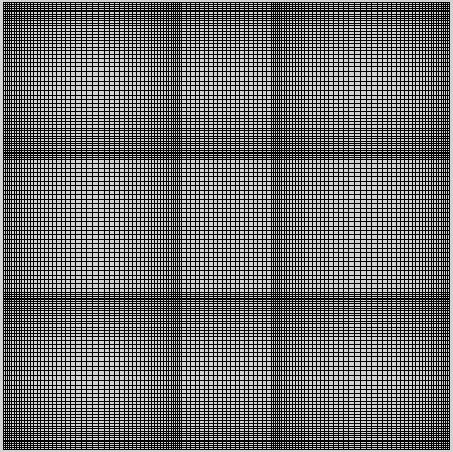## 3b.jpgFigure 3. Grid validation using non-uniform mesh and average Nu with different number of nodes

The Eddie present at the top seems to get flattered with size enlargement by increasing Ha. Hence the circulation of fluid from the heater to the cold moving wall is getting disturbed. At Ri=0.01 the isotherm lines closer to the cold moving wall are flatter and thus showing the entrapment of the hot fluids which leads to more heat transport to the cold wall of the enclosure with a vertical rectangle as compared to the other shapes. As a result, maximum heat transfer is taking place with a vertical rectangle at each Ha among all shapes. For Ri=10 it can be seen from the streamlined contour that for a given shape as Ha is increasing, the size of the cavity present at the bottom on the right-hand side and circulating around the obstacle is increasing. This phenomenon is highly governed by the natural free convection. With the increase in cavity size, it provides more restriction to heat flow taking place from bottom to top and resulting in a reduction of heat transport to the upper wall.

At Ri=0.01, only one Eddie can be seen near the top wall. But in the case of Ri=10, three eddies are present. This is due to the enhancement in thermal expansion coefficient (β) at Ri=10. and leads to higher Nu at the upper wall for higher Ri as the velocity of the lid is constant at both the Ri.

## 4a.jpg## 4b.jpgFigure 4. Streamlines and isotherms contours for constant Ri=0.01 and φ=0 with varying Ha and shape of the obstacles

The rise in β at lower Ri results in dominance of the free convection. At Ri=0.01 it can be noted that isotherm lines transferring the heat from the bottom wall mainly heating the left part of the cold moving wall. This results in differential heating of the cold moving wall. The numerical analysis performed at both the Ri shows that due to the applied magnetic field as Ha increases flow stabilization takes place due to which a particular isotherm line gets more and more deviated from reaching the cold wall. This results in the reduction of avg. Nu at the cold moving wall.

Figure 6 shows the comparison of streamline patterns at a particular Hartmann number (Ha=50) to show the variation in heat flow with increase in nanofluid volume fraction. It can be seen that at a particular Ri streamline patterns are almost identical at all φ for the different shape of the obstacle which implies that the effect of the nanofluid is very negligible in presence of magnetic field.

## 5a.jpg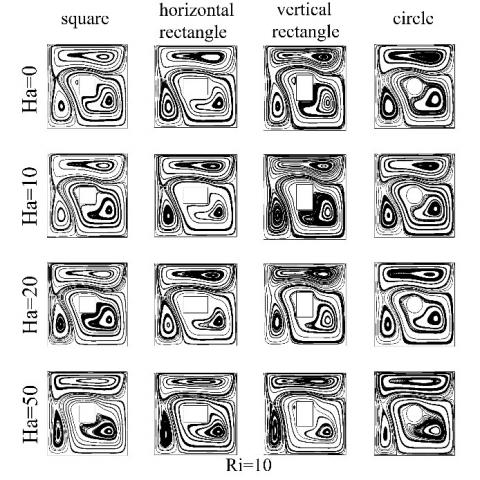## 5b.jpg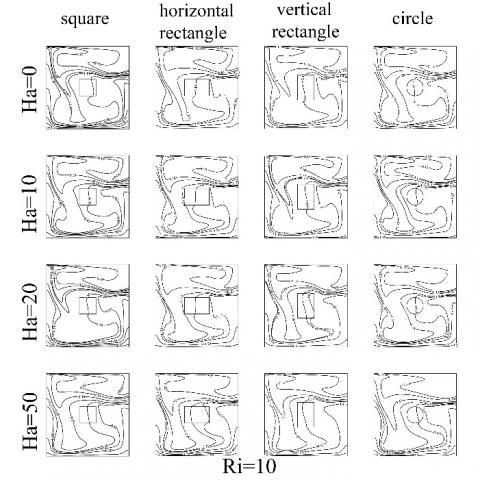Figure 5. Streamline and isotherms for constant Ri=10 and φ=0 with varying Ha and shape of the obstacles

Figure 7 shows the streamline contours to analyze the heat transfer phenomenon with variation in Ha as well as φ at a particular shape of the obstacle. It is observed that for a particular shape with an increase in Ha the Eddie present at the top is getting bigger which results in the reduction of heat transfer but with an increase in φ streamline patterns remains almost identical. This shows that the MHD flow predominates the effect of φ.

## 6.jpg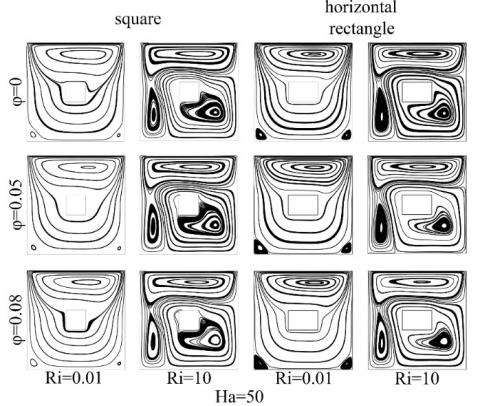Figure 6. Streamline at different Ri, Ha and φ for the horizontal rectangle and square shaped obstacles

## 7.jpgFigure 7. Streamline at different Ri, Ha, and φ for the vertical rectangle shaped obstacle

## 8.jpgFigure 8. Variations in streamline and isotherm contours without considering MHD

Figure 8 shows streamlines and isotherms for the square obstacle without the application of MHD. At Ri=0.01 it can be noted that isotherm lines transferring the heat from the bottom wall mainly heating the left part of the cold moving wall. This results in differential heating of the cold moving wall. The numerical analysis performed at both Ri shows that as φ increases the heat transport capacity of nanofluid enhances because the conductivity of base fluid rises with the addition of nano-materials. This results in the increment of avg. Nu at the cold moving wall.

To distinguish heat transfer in any system Nu is the key parameter to analyze the efficiency of the heat transport phenomena. Figures 9 and 10 show the average Nu variation on the cold wall for different shaped obstascles at Ri 0.01 and 10 respectively. Average Nu decreases with the increase in Ha due to the flow stabilization. Increased thermal expansion coefficient results an increase in the value of heat transfer at higher Ri.

## 9.jpgFigure 9. Nuavg. of the cold wall for various shaped obstacles at Ri=0.01

## 10.jpg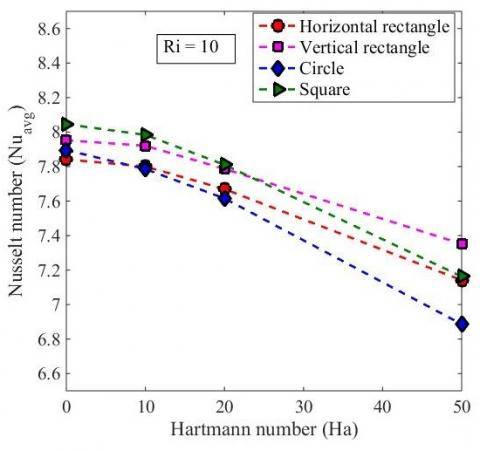Figure 10. Nuavg. of the cold wall for various shaped obstacles at Ri=10

4. Conclusions

The study conducted illustrates the effect of governing parameters on the thermal and flow field using MHD. The motion of the lid, heaters and applied magnetic field are responsible for changes in heat transfer inside the cavity and few concluding statements can be put forward as:

• For each shape of obstacle the Nuavg at the cold wall decreases as the Ha increases.
• The Richardson number variation is obtained by changing the thermal expansion coefficient. At Ri=0.01, in case of a vertical rectangle, maximum Nu is obtained for all Ha as the increase in the horizontal flow field is maximum in case of a vertical rectangle. At Ri=10, due to the increase in thermal expansion coefficient the maximum Nuavg is obtained in the case of a square rectangle.
• MHD flow predominates the effect of percentage volume of nanoparticles for a particular shape of obstacle inside the cavity.
Nomenclature
 B0           Magnetic field strength [kg. s-2. A-1] Cp         Specific heat at constant pressure [J. kg-1. K] g           Gravitational acceleration [m. s-2] Re         Reynolds number Ha         Hartmann number J            Joule heating parameter K          Thermal conductivity [W. m-1. K-1] Gr         Grashof number L           Length of the square enclosure [m] N           Interaction parameter Nu         Nusselt number Pr           Prandtl number Ri           Richardson number T            Temperature [K] u1,u2      Velocity components [m. s-1] U1,U2     Dimensionless velocity components U0          Lid velocity [m. s-1] x1,x2      Cartesian coordinates [m] X1,X2     Dimensionless Cartesian coordinate Greek symbols µ             dynamic viscosity [kg. m-1. s-1] α             Thermal diffusivity [m-2. s] β             Thermal expansion coefficient [K-1] η             Normal direction θ             Dimensionless temperature ρ             Density of fluid [kg. m-3] σ             Electrical conductivity [S. m-1] υ             Kinematic viscosity of the fluid [m2. s-1] Subscripts avg.        average c             cold f              fluid h              hot s              solid
References

 Rahman, M.M., Alim, M.A., Sarker, M.M.A. (2010). Numerical study on the conjugate effect of Joule heating and magneto-hydrodynamics mixed convection in an obstructed lid-driven square cavity. International Communications in Heat and Mass Transfer, 37(5): 524-534. https://doi.org/10.1016/j.icheatmasstransfer.2009.12.012

 Ray, S., Chatterjee, D. (2014). MHD mixed convection in a lid-driven cavity including a heat conducting circular solid object and corner heaters with Joule heating. International Communications in Heat and Mass Transfer, 57: 200-207. https://doi.org/10.1016/j.icheatmasstransfer.2014.07.029

 Saha, L.K., Somadder, M.C., Roy, N.C. (2015). Hydro-magnetic mixed convection flow in a lid-driven cavity with the wavy bottom surface. American Journal of Applied Mathematics, 3(1): 8-19. http://dx.doi.org/10.11648/j.ajam.s.2015030101.12

 Farid, S.K., Billah, M.M., Rahman, M.M., Sharif, U.M. (2013). Numerical study of fluid flow on magneto-hydrodynamic mixed convection in a lid-driven cavity having a heated circular hollow cylinder. Procedia Engineering, 56: 474-479. http://dx.doi.org/10.1016/j.proeng.2013.03.149

 Mahalakshmi, T., Nithyadevi, N., Oztop, H.F., Abu-Hamdeh, N. (2018). MHD mixed convective heat transfer in a lid-driven enclosure filled with Ag-water nanofluid with a center heater. International Journal of Mechanical Sciences, 142: 407-419. http://dx.doi.org/10.1016/j.ijmecsci.2018.05.008

 Oztop, H.F., Ahmed, S., Abu-Nada, E., Al-Salem, K. (2017). Mixed convection of MHD flow in nanofluid filled and partially heated wavy walled lid-driven enclosure. International Communications in Heat and Mass Transfer, 86: 42-51. https://doi.org/10.1016/j.icheatmasstransfer.2017.05.011

 Selimefendigil, F., Oztop, H.F. (2016). Analysis of MHD mixed convection in a flexible-walled and nanofluids filled a lid-driven cavity with volumetric heat generation. International Journal of Mechanical Sciences, 118: 113-124. https://doi.org/10.1016/j.ijmecsci.2016.09.011

 Hussain, S., Mehmood, K., Sagheer, M. (2016). MHD mixed convection and entropy generation of water–alumina nanofluid flow in a double lid-driven cavity with discrete heating. Journal of Magnetism and Magnetic Materials, 419: 140-155. https://doi.org/10.1016/j.jmmm.2016.06.006

 Gangawane, K.M., Oztop, H.F., Abu-Hamdeh, N. (2018) Mixed convection characteristic in a lid-driven cavity containing heated triangular block: Effect of location and size of the block. International Journal of Heat and Mass Transfer, 124: 860-875. https://doi.org/10.1016/j.ijheatmasstransfer.2018.03.079

 Mehmood, K., Hussain, S., Sagheer, M. (2017). Mixed convection in alumina-water nanofluid filled lid-driven square cavity with an isothermally heated square blockage inside with magnetic field effect. International Journal of Heat and Mass Transfer, 109: 397-409. https://doi.org/10.1016/j.ijheatmasstransfer.2017.01.117

 Zhou, W., Yan, Y., Liu, X., Chen, H., Liu, B. (2018). Lattice Boltzmann simulation of mixed convection of nanofluid with different heat sources in a double lid-driven cavity. International Communications in Heat and Mass Transfer, 97: 39-46. https://doi.org/10.1016/j.icheatmasstransfer.2018.07.008

 Sharma, B., Kumar, B., Barman, R.N. (2018). Numerical investigation of Cu-water nanofluid in a differentially heated square cavity with conducting solid square cylinder at center. International Journal of Heat Technology, 36: 714-722. https://doi.org/10.18280/ijht.360238

 Bora, M.K., Alam, M.F., Sharma, B., Barman, R.N. (2019). Numerical investigation of Ag-H2O nanofluid in a lid driven square cavity with different shaped conducting and insulating cylinders placed at centre. International Journal of Heat and Technology, 37(3): 831-838. https://doi.org/10.18280/ijht.370320

 Fluent, Ansys12.0 Theory Guide. Ansys Inc. (2009).

 Maxwell, J.C. (1873). Electricity and Magnetism Clarendon PressReber, E.E., Michell, R.L., Carter, C.J. (1988).